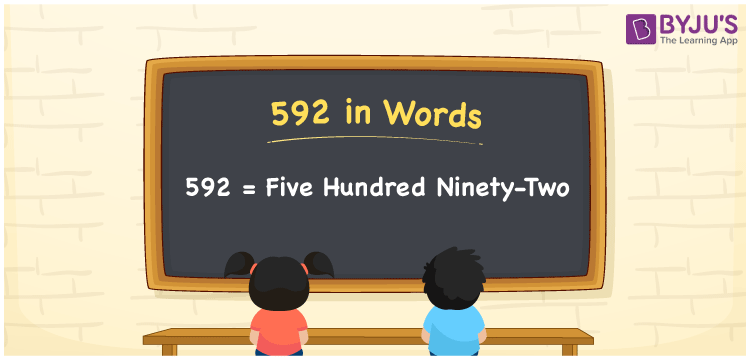# 592 in words

592 in words is written as Five Hundred and Ninety Two. 592 represents the count or value. The article on Counting Numbers can give you an idea about count or counting. The number 592 is used in expressions that relate to money, distance, length, year and others. Let us consider an example for 592. ”Can you read a novel that has Five Hundred and Ninety Two pages in it?”

 592 in words Five Hundred and Ninety Two Five Hundred and Ninety Two in Numbers 592

## 592 in English Words## How to Write 592 in Words?

We can convert 592 to words using a place value chart. The number 592 has 3 digits, so let’s make a chart that shows the place value up to 3 digits.

 Hundreds Tens Ones 5 9 2

Thus, we can write the expanded form as:

= 5 × Hundred + 9 × Ten + 2 × One

= 5 × 100 + 9 × 10 + 2 × 1

= 592

= Five Hundred and Ninety Two.

592 is the natural number that is succeeded by 591 and preceded by 593.

592 in words – Five Hundred and Ninety Two.

Is 592 an odd number? – No.

Is 592 an even number? – Yes.

Is 592 a perfect square number? – No.

Is 592 a perfect cube number? – No.

Is 592 a prime number? – No.

Is 592 a composite number? – Yes.

## Solved Example

1. Write the number 592 in expanded form

Solution: 5 × 100 + 9 × 10 + 2 × 1

We can write 592 = 500 + 90 + 2

= 5 × 100 + 9 × 10 + 2 × 1

## Frequently Asked Questions on 592 in words

### How to write 592 in words?

592 in words is written as Five Hundred and Ninety Two.

### Is 592 a perfect square number?

No. 592 is not a perfect square number.

### Is 592 a prime number?

No. 592 is not a prime number.